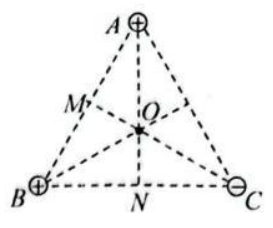$\text{A.}$ $M, N$ 两点的电场强度大小相等 $\text{B.}$ $M$ 点的电势高于 $N$ 点的电势 $\text{C.}$ 将一个不计重力的负电荷沿直线由 $O$ 点向 $C$ 点移动的过程中, 电势能逐渐减小 $\text{D.}$ 将一个不计重力的负电荷沿直线由 $O$ 点向 $N$ 点移动的过程中, 受到的电场力做负功

BD
①点击 收藏 此题， 扫码注册关注公众号接收信息推送（一月四份试卷,中1+高2+研1）
② 程序开发、服务器资源都需要大量的钱，如果你感觉本站好或者受到到帮助，欢迎赞助本站,赞助方式：微信/支付宝转账到 18155261033

 ①此题难易度如何 ②此题推荐度如何 确定